## Friday, January 18, 2019

### MCCR test for Pybus & Harvey's γ on incompletely sampled trees using phytools

The MCCR test for Pybus & Harvey's (2000) γ statistic from incompletely sampled phylogenies used to be implemented in the no-longer-available-on-CRAN laser package of Dan Rabosky. The method is pretty simple, though, & since we use it in teaching I figured it would be easy enough to add to phytools.

Here is a function to do the test, as well as `print` & `plot` methods for the resultant object:

``````mccr<-function(obj,rho=1,nsim=100,plot=TRUE){
N<-round(Ntip(obj\$tree)/rho)
tt<-pbtree(n=N,nsim=nsim)
foo<-function(x,m) drop.tip(x,sample(x\$tip.label,m))
tt<-lapply(tt,foo,m=N-Ntip(obj\$tree))
g<-sapply(tt,function(x) ltt(x,plot=FALSE)\$gamma)
P<-if(obj\$gamma>median(g)) 2*mean(g>=obj\$gamma) else 2*mean(g<=obj\$gamma)
result<-list(gamma=obj\$gamma,"P(two-tailed)"=P,null.gamma=g)
class(result)<-"mccr"
result
}

print.mccr<-function(x,digits=4,...){
cat("Object of class \"mccr\" consisting of:\n\n")
cat(paste("(1) A value for Pybus & Harvey's \"gamma\"",
" statistic of ",round(x\$gamma,digits),".\n\n",sep=""))
cat(paste("(2) A two-tailed p-value from the MCCR test of ",
round(x\$'P(two-tailed)',digits),".\n\n", sep = ""))
cat(paste("(3) A simulated null-distribution of gamma from ",
length(x\$null.gamma)," simulations.\n\n",sep=""))
}

plot.mccr<-function(x,...){
hist(x\$null.gamma,breaks=min(c(max(12,round(length(x\$null.gamma)/10)),20)),
xlim=range(c(x\$gamma,x\$null.gamma)),
main=expression(paste("null distribution of ",
gamma)),xlab=expression(gamma),col="lightgrey")
arrows(x0=x\$gamma,y0=par()\$usr,y1=0,length=0.12,
col=make.transparent("blue",0.5),lwd=2)
text(x\$gamma,0.98*par()\$usr,
expression(paste("observed value of ",gamma)),
pos=if(x\$gamma>mean(x\$null.gamma)) 2 else 4)
}
``````

Now let's see how to use it. First, I'll run it on the completely sampled tree:

``````## pure birth tree with complete sampling:
pb<-pbtree(n=100)
lineages<-ltt(pb)
``````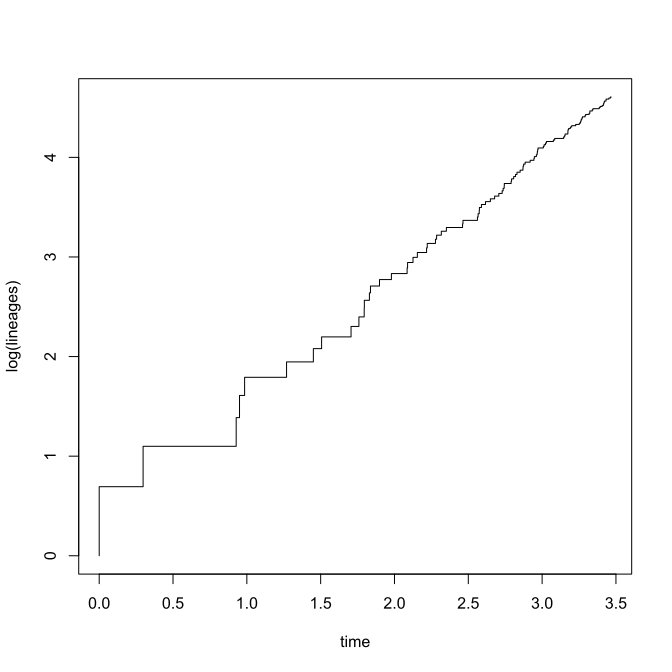``````lineages
``````
``````## Object of class "ltt" containing:
##
## (1) A phylogenetic tree with 100 tips and 99 internal nodes.
##
## (2) Vectors containing the number of lineages (ltt) and branching times (times) on the tree.
##
## (3) A value for Pybus & Harvey's "gamma" statistic of -0.2688, p-value = 0.7881.
``````
``````## MCCR test assuming complete sampling:
object<-mccr(lineages,rho=1,nsim=200)
object
``````
``````## Object of class "mccr" consisting of:
##
## (1) A value for Pybus & Harvey's "gamma" statistic of -0.2688.
##
## (2) A two-tailed p-value from the MCCR test of 0.67.
##
## (3) A simulated null-distribution of gamma from 200 simulations.
``````
``````plot(object)
``````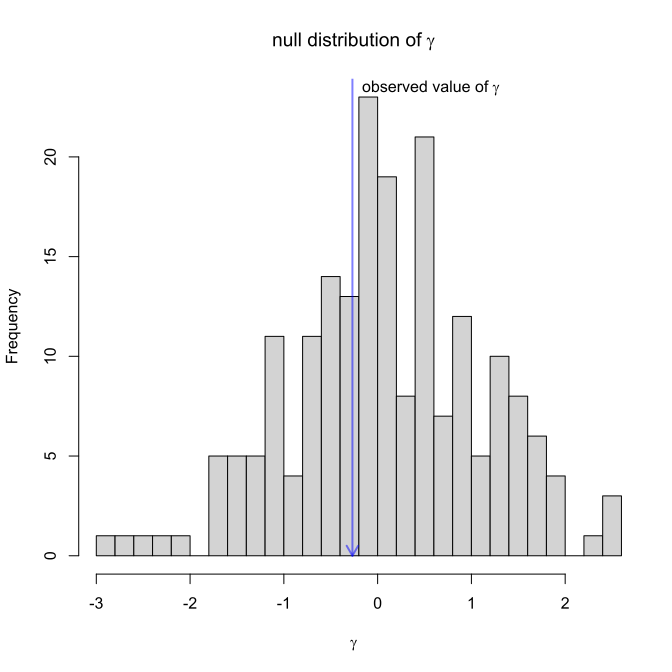A neat thing to show is that the MCCR test (based on simulation) will give a P-value that converges on the P-value from the parametric test if you run enough simulations. Let's see that:

``````object<-mccr(lineages,rho=1,nsim=10000)
lineages
``````
``````## Object of class "ltt" containing:
##
## (1) A phylogenetic tree with 100 tips and 99 internal nodes.
##
## (2) Vectors containing the number of lineages (ltt) and branching times (times) on the tree.
##
## (3) A value for Pybus & Harvey's "gamma" statistic of -0.2688, p-value = 0.7881.
``````
``````object
``````
``````## Object of class "mccr" consisting of:
##
## (1) A value for Pybus & Harvey's "gamma" statistic of -0.2688.
##
## (2) A two-tailed p-value from the MCCR test of 0.7942.
##
## (3) A simulated null-distribution of gamma from 10000 simulations.
``````

Next, we can simulate incomplete sampling, see the effect on the P-value from `ltt`, and then compare that to a P-value obtained from the MCCR test:

``````incomplete.pb<-drop.tip(pb,sample(pb\$tip.label,round(0.5*Ntip(pb))))
lineages<-ltt(incomplete.pb,plot=FALSE)
lineages
``````
``````## Object of class "ltt" containing:
##
## (1) A phylogenetic tree with 50 tips and 49 internal nodes.
##
## (2) Vectors containing the number of lineages (ltt) and branching times (times) on the tree.
##
## (3) A value for Pybus & Harvey's "gamma" statistic of -0.9876, p-value = 0.3234.
``````
``````object<-mccr(lineages,rho=0.5,nsim=200)
object
``````
``````## Object of class "mccr" consisting of:
##
## (1) A value for Pybus & Harvey's "gamma" statistic of -0.9876.
##
## (2) A two-tailed p-value from the MCCR test of 0.76.
##
## (3) A simulated null-distribution of gamma from 200 simulations.
``````

Finally, for fun we can simulate a tree with a shape that should correspond to a positive value of γ. Here, I'll do it using the phytools internal function `ebTree`. We can then subsample the tree to simulate incomplete sampling.

``````eb<-phytools:::ebTree(pbtree(n=200,scale=1),-1.2)
ltt(eb)
``````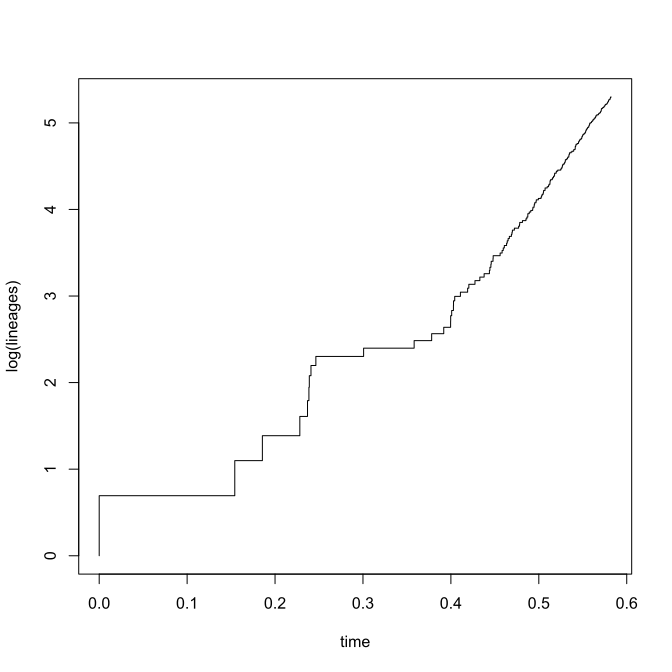``````## Object of class "ltt" containing:
##
## (1) A phylogenetic tree with 200 tips and 199 internal nodes.
##
## (2) Vectors containing the number of lineages (ltt) and branching times (times) on the tree.
##
## (3) A value for Pybus & Harvey's "gamma" statistic of 2.0067, p-value = 0.0448.
``````
``````incomplete.eb<-drop.tip(eb,sample(eb\$tip.label,round(0.5*Ntip(eb))))
ltt(incomplete.eb)
``````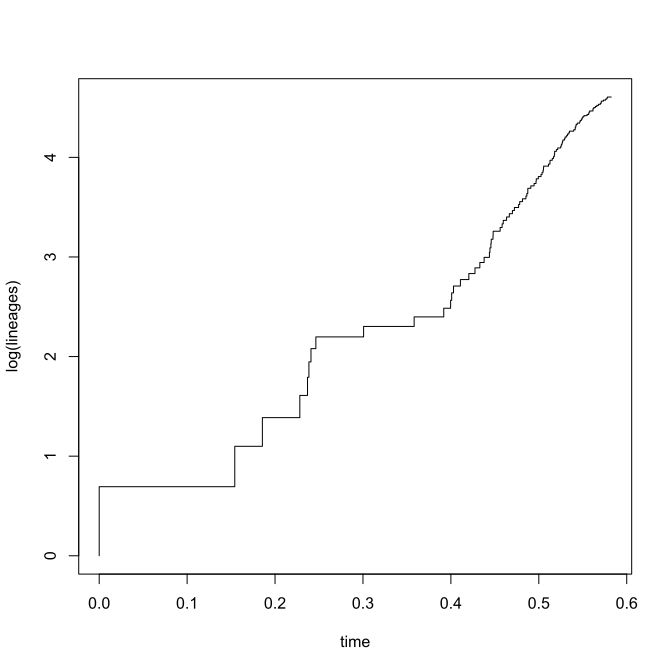``````## Object of class "ltt" containing:
##
## (1) A phylogenetic tree with 100 tips and 99 internal nodes.
##
## (2) Vectors containing the number of lineages (ltt) and branching times (times) on the tree.
##
## (3) A value for Pybus & Harvey's "gamma" statistic of -0.2614, p-value = 0.7938.
``````
``````object<-mccr(ltt(incomplete.eb,plot=FALSE),rho=0.5,nsim=200)
object
``````
``````## Object of class "mccr" consisting of:
##
## (1) A value for Pybus & Harvey's "gamma" statistic of -0.2614.
##
## (2) A two-tailed p-value from the MCCR test of 0.06.
##
## (3) A simulated null-distribution of gamma from 200 simulations.
``````
``````plot(object)
``````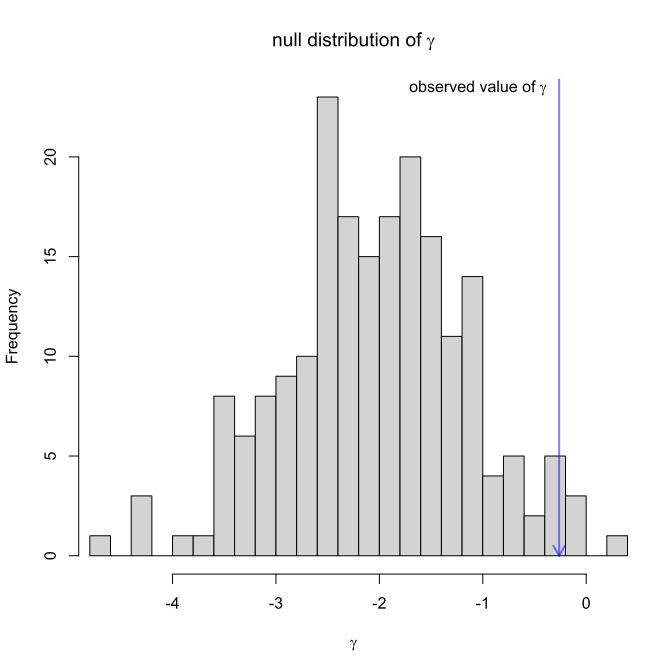Neat.

This is not yet in phytools, but I will add documentation & put it in a future release.